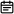# 解决了！微信公众号数学公式排版2019-10-14

https://mdnice.com/ 给你最完美的解决方案！## 行间公式

\begin {aligned} c (\theta{1}) C (\theta{2}) =&(\cos \theta{1}+i \sin \theta{1})\left (\cos \theta{2}+i \sin \theta{2}\right) \ =&\left{\left (\cos \theta{1} \cos \theta{2}-\sin \theta{1} \sin \theta{2}\right)\right.\ &i\left (\cos \theta{1} \sin \theta{2}+\cos \theta{2} \sin \theta{1}\right)} \ =&\cos \left (\theta{1}+\theta{2}\right)+i \sin \left (\theta{1}+\theta{2}\right) \ =&c\left (\theta{1}+\theta{2}\right) \end {aligned}

$$\left|z{1}+z{2}+\cdots+z{n}\right| \leqslant\left|z{1}\right|+\left|z{2}\right|+\cdots+\left|z{n}\right|.$$

$$\begin{pmatrix} 1 & a1 & a1^2 & \cdots & a1^n \ 1 & a2 & a2^2 & \cdots & a2^n \ \vdots & \vdots & \vdots & \ddots & \vdots \ 1 & am & am^2 & \cdots & a_m^n \ \end{pmatrix}$$

## 使用方法1、行内公式：将公式前后各加 1 个 $ 符号，中间插入 LaTeX 语法。 一般情况下$a+b$的结果 2、行间公式：将公式前后各加 2 个 $ 符号，中间插入 LaTeX 语法。

$$\begin{pmatrix} 1 & a_1 & a_1^2 & \cdots & a_1^n \\ 1 & a_2 & a_2^2 & \cdots & a_2^n \\ \vdots & \vdots & \vdots & \ddots & \vdots \\ 1 & a_m & a_m^2 & \cdots & a_m^n \\ \end{pmatrix}$$1. \tag{xxx} 改为 \qquad (xxx)（避免公式被缩小）
2. 长的行内公式改为行间公式（优化断行），并适当换行。不要直接使用 \\ 来换行，要使用 aligned 等对其环境。（避免公式被缩小）